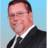Dialog testing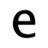I am playing around with the dialog() function to figure out how it works. I created a little app that can be used to give a quiz, or take a survey. Right now it just records results into a text field, but it should be something else. This is just so I can play around.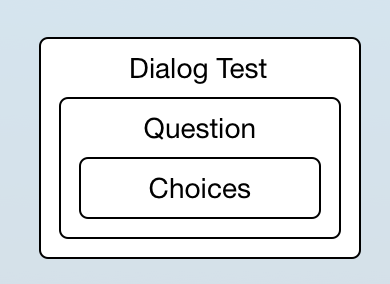When you click the button on the Dialog Test form, a dialog() will pop up for each Question.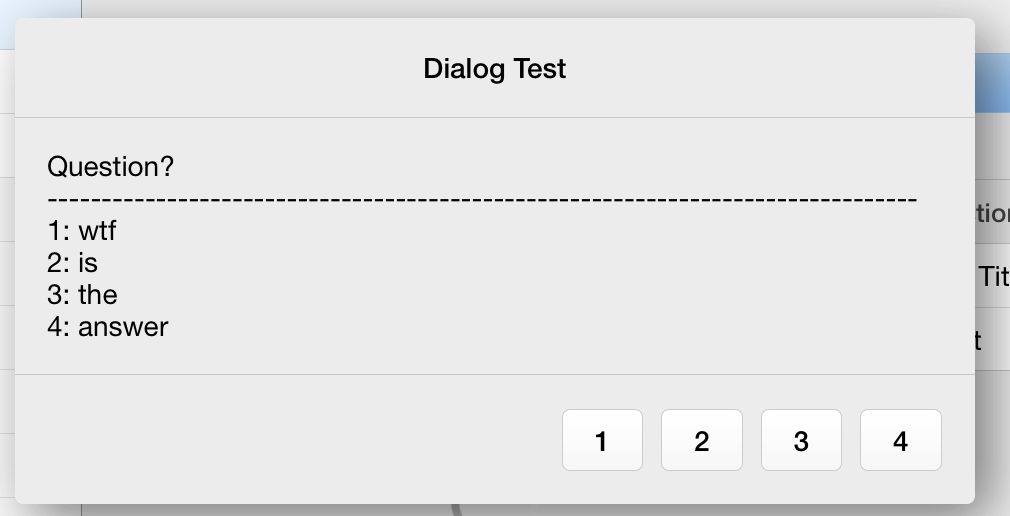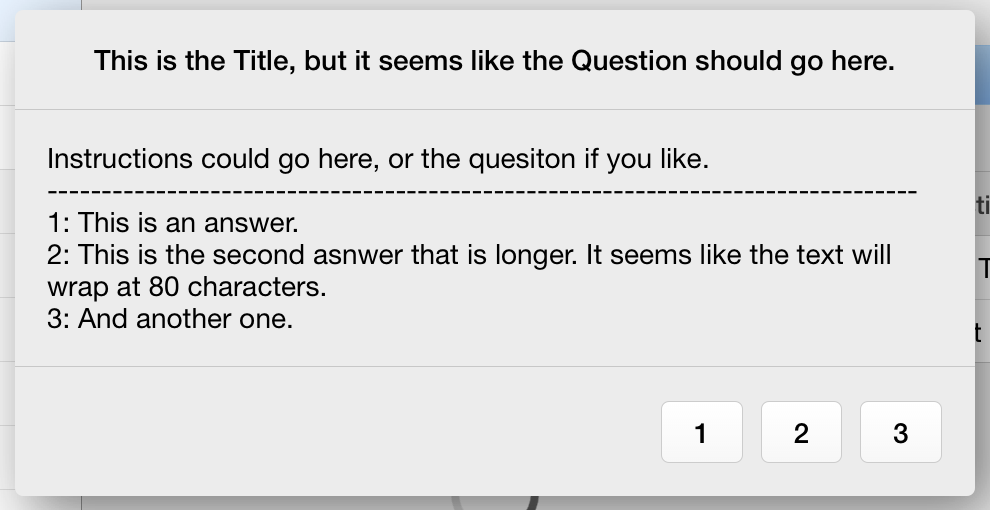This is the button code on the Dialog Test form:

let Main := this;
for i in Question.Instructions do
let Questions := text(unique(Question.[i]));
let L := length(Questions);
let QP := 112;
if L > 80 then QP := L + 80 end;
let QA := rpad(Questions, QP, " ") + rpad("-", 80, "-") + " ";
for ii in Question[Instructions = i].Choices.AnswerID do
L := length(A);
if L > 80 then QP := L + 80 end;
QA := QA + rpad(text(unique(ii)) + ": " + A, QP, " ")
end;
let response := dialog(text(unique(Question[Instructions = i].Question)), QA, Question[Instructions = i].Choices.AnswerID);
Text := Text + response
end

A few things I have noticed so far.

Choices for answers should be limited to 10. 11 will fit, but doesn't look quite right. 12 will be over the edge of the dialog window.

The iPhone version of Ninox does not display dialog() the same way the OSX or web version of the app does. My code doesn't look right on the iPhone. I assume it is the same on the iPad, but I haven't tried it yet.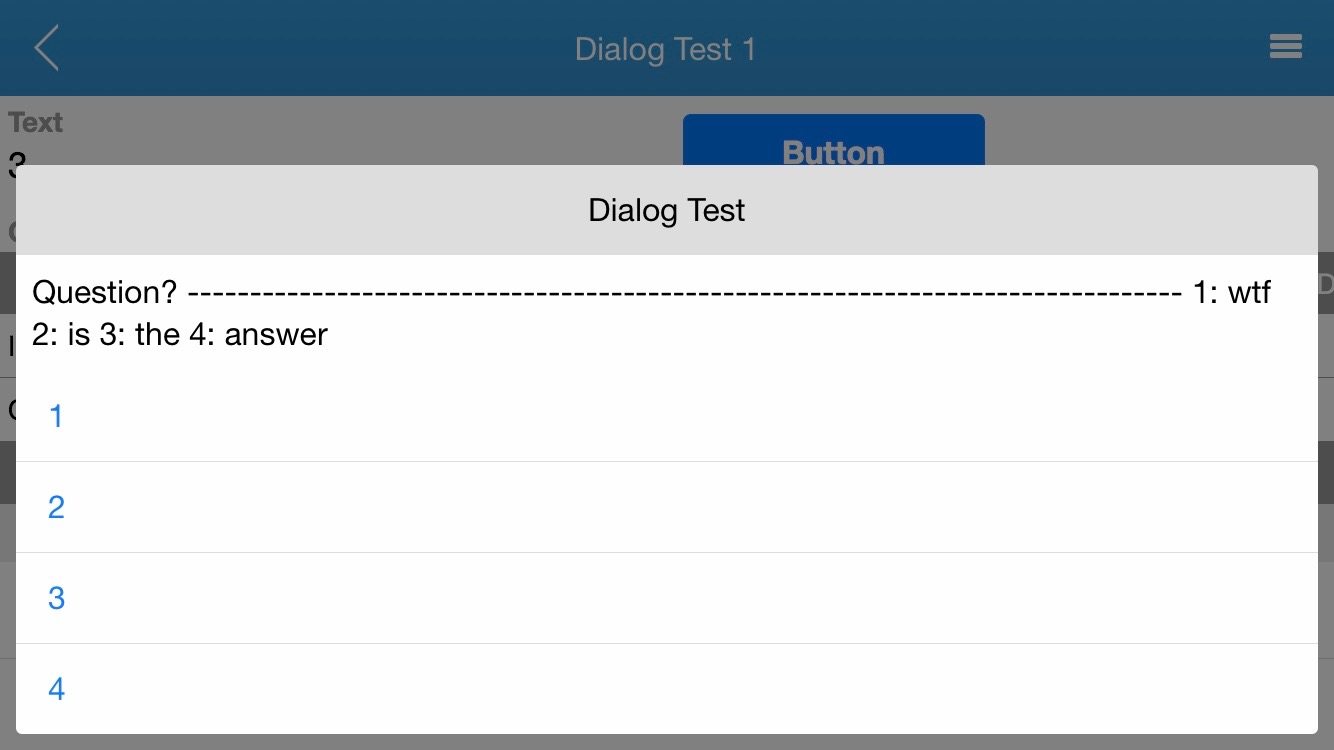I also noticed that some code does not work if the DB is hosted local. This is the code I use to automagicly create the AnswerID in the Choices record. It will work if the DB is on Ninox Cloud, but will not work if the DB is local or on iCloud.